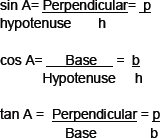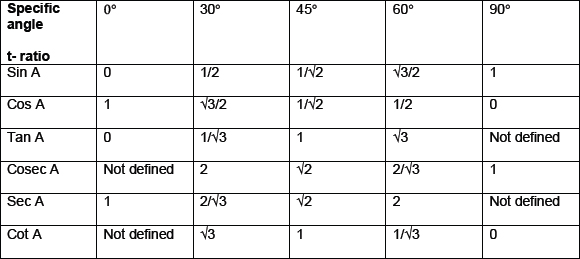# CBSE Class 10 Math, Introduction to Trigonometry

INTRODUCTION TO TRIGONOMETRY

KEYNOTES
Trigonometric ratios
The certain ratios involving the sides of a right angled triangle are called Trigonometric ratios.
Suppose: b is the base
h is the hypotenuse
p is perpendicular
then,Reciprocals of the ratios are:
Cosec A= 1/sin A= h/p
Sec A= 1/cos A= h/b
Cot A= 1/tan A= b/p
Sin □ is a single symbol and sin cannot be detached from ‘□’. And sin □ ≠ sin X □.
This remark is true for other ratios as well
Trigonometric /Ratios of some specific angles
The specific angles are 0°, 30°,45°, 60°, 90°. These are given in the following tableThe value of sin A increases from 0 to 1, as A increases from 0° to 90°
The value of cosA decreases from 1 to 0, as A increases from 0° to 90°
The value of tan A increases from 0 to infinity, as A increases 0° to 90°
√2 = 1.414 and √3 = 1.732
Trignometric identitiex
Cos2 A+ sin2 A = 1
1+tan2 A= sec2 A
Cot2 A+1= cosec2 A
Trigonometric ratios of complementary angles
Two angles are said to be complementary if their sum equals to 90°
1. Sin (90°-A)= cos A
2. Cos (90°-A)= sin A
3. Tan (90°-A)= cotA
4. Cot (90°-A)= tan A
5. Sec (90°- A)= cosec A
6. Cosec( 90°- A)= sec A
Note
Tan 0°= cot 90°= 0
Sec0°=cosec 90°=1
Sec 90°, cosec 0°, cot 0° and tan 90° are not defined